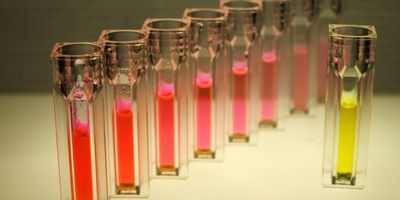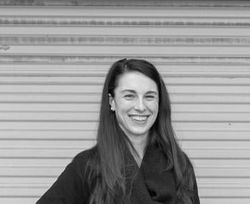## InsightsiStock, pjelev

# How to Make a Calibration Curve

## A step-by-step guide

Michelle Dotzert, PhDMichelle Dotzert, PhD

Michelle Dotzert is the creative services manager for Lab Manager. She holds a PhD in Kinesiology (specializing in exercise biochemistry) from the University of Western Ontario. Her research examined the...

View

## Calibration curves

This guide will describe the process for preparing a calibration curve, also known as a standard curve.

## What is a calibration curve?

Calibration curves are often used in many fields, including analytical chemistry and biochemistry. A calibration curve is used to determine the concentration of an unknown sample, to calculate the limit of detection, and the limit of quantitation. The curve is created from the instrumental response to a set of standard samples at a range of concentrations. The data are then fit with a function to enable the prediction of unknown concentrations.### What is an ultraviolet-visible (UV-Vis) spectrophotometer?

A UV-vis spectrophotometer is an instrument used to measure the transmission and absorption of light to determine the concentration of an analyte in solution.

The UV-Vis spectrophotometer consists of a light source, a wavelength selector, a detector, and a computer. The light source may be a single xenon lamp, or a tungsten or halogen lamp and a deuterium lamp. The wavelengths of light required for analyte detection may be selected using a monochromator or a variety of different filters.### How does a UV-Vis spectrophotometer work?

The UV-Vis light passes through the sample and reaches the detector. The transmittance is measured and used to calculate the absorbance. An absorption spectrum is obtained with absorbance plotted on the vertical y-axis and wavelength plotted on the horizontal x-axis.## What you will need to make a calibration curve:

• Personal protective equipment (gloves, lab coat, eye protection, etc.)
• Standard solution
• Solvent
• Pipette and tips
• UV-Vis spectrophotometer
• Cuvettes
• Computer## Step 1: Make a concentrated stock solution

Prepare a concentrated stock solution of the standard by weighing the solute and transferring it to a volumetric flask with solvent.
See this step-by-step guide for making aqueous solutions.

## Step 2: Make the standards for the calibration curve

### Perform a serial dilution

Label a series of volumetric flasks or microtubes. A minimum of five standards are recommended for a good calibration curve.

Pipette the required volume of standard into the first flask or microtube. Change the pipette tip, add the required volume of solvent to the same flask or microtube, then mix.

Repeat this process by pipetting from the previous solution to the new flask or microtube and adding solvent.

For more details, see the step-by-step guide to serial dilutions.### Prepare the samples and unknowns

Transfer the standards to cuvettes.

Transfer the unknown samples to cuvettes. The unknown samples should have the same buffer and pH as the standards.## Step 3: Run the standards and samples in the spectrophotometer

Place each standard in the UV-Vis spectrophotometer and obtain a reading. Obtain between three and five readings for each standard and record the data in a spreadsheet.

Repeat with the unknown samples.## Step 4: Plot the data

Plot the data with absorbance on the y-axis and concentration on the x-axis.

If samples were measured in triplicate, use this data to determine the standard deviation and add error bars.

## Step 5: Examine the calibration curve

Examine the plot. The calibration curve should look linear and have a section that is non-linear—this is the limit of linearity (LOL), a sign that instrumental detection is nearing saturation.### Fit the data to a linear regression

Use statistical software to fit the data to a linear regression.

The output is the following equation:

y = mx + b

Where m is slope (the units are absorbance/µm), and b is the y-intercept (the units are absorbance).### Obtain a coefficient of determination (R2)

The coefficient of determination (R2) quantifies goodness of fit—the square of the correlation coefficient between actual and predicted Y values. R2 is typically a fraction between 0.0 and 1.0, with 1.0 being a perfect fit.

Tags: